I.2.1

2/1/2021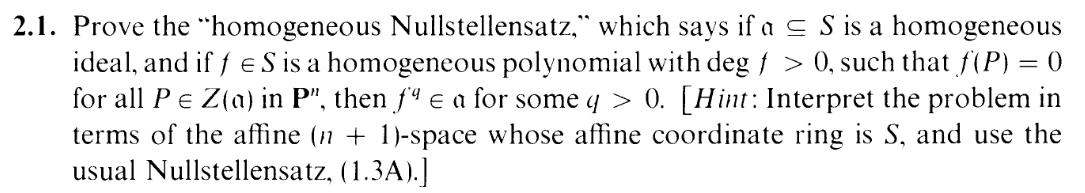(S = k[x0,x1,,xn] in these exercises).

I'm sorry for the hiatus. I've been extremely busy lately. Well, not really. But you know... you're used to these kind of gaps, right? By this point it's par the course. Oh? I see, I see, you missed me. You'd THINK you would have gotten used to it by now, but each time I go on these outings, you can't help but feel the pain of my absence. Shuffling about the house, daydreaming about my face. Lying in bed, alone, with only a picture of me at the bedside to soothe the loneliness a bit. You look at my photographed subtle smile, wishing I was there with you, noticing the blemish on my cheek, blushing a bit, and, feeling a little guilty, your hand slowly crawls down towards your nether regions...

Anyway, this exercise is supposed to be very simple, but it confused the ever living fuck out of me. The main issue is getting confused about whether I'm working in projective or affine space, especially in an exercise that involves both. A good mathematician, given a "point" P = (x0,x1,,xn) would be able to interchangeably use this "P" in both projective and affine space and understand where he's at (wombo combo hurr durr). In fact, this is what the book does. HOWEVER, I suck. And trying to be clever like this fucked me in the ass. So let's not be clever and make things explicit.

What is projective space? Pn is just (An+1 - 0)~ where (x0,x1,,xn) ~ (cx0,cx1,,cxn) where c0. Letting π : (An+1 - 0) Pn be the quotient morphism, I'll denote π(P) = [P]. So, e.g. a point Q Pn is actually Q = [P] for a point P An+1 - 0, where [P] = [cP]. PHEW. *Wipes forehead*.

One more thing to get things explicit: I'll reframe another definition in the book in this notation. That is, for a homogenous polynomial f S and [P] Pn, we define: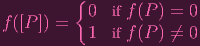(1)

I.E., for P An+1 where P0, we have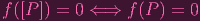(2)

FUCKING. PHEW. *WIPES.* *FOREHEAD.* (had to figure out how to use the \cases environment again)

Now that we've established that cancer. let's unravel the "hint". Let's compute the affine coordinate ring of An+1 in An+1. I.e. A(An+1) = k[x0,x1,,xn]∕I(An+1) =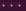This is getting tedious= S∕I(An+1) = S∕(0) = S. *WIPES MOTHERFUCKING FOREHEAD AGAIN*. (I should already know that the coordinate ring of An+1 is S. In fact, I had a whole fucking speech about it in an earlier post. Woops).

Okay, now are we ready to rumble? The point is that Pn and An+1 share the same coordinate ring, S, so we can use the normal Nullstellensatz (below) to prove this so-called "homogenous Nullstellensatz".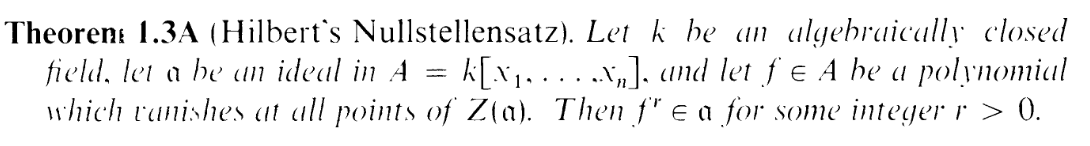Now suppose α is a homogenous ideal. I.e. it is generated by homogenous elements which I'll denote f1,f2, (actually, according to the book because of Noetheriannness it's generated by finitely many homog elements, but there's no need to use that fact for this exercise. I'll keep it general). And suppose we have f as given in the exercise. We need to show fq α for some q > 0.

Okay... one more "expletive" (FUCK!) to make this clear:

When we say Z(α) we could either be taking the zero set in projective or affine space. So when I'm taking it in projective space, I'll denote it ZP and for affine space I'll say ZA (don't worry, I am using macros to not turn this into the syntax olympics). so the f we're given here satisfies f(P) = 0,P ZP(α). To apply the regular Nullstellensatz we basically want to replace the ZP with ZA.
Okay, NOW, we're done with the explicitizing. *FOREHEAD WIPE MARK IV*

CONSIDER, dear reader, an element Q from ZA(α).

CASE 1: Q0:
A point being in the zero set of an ideal is equivalent to it being zero on the ideal's generators. I.e. we have f1(Q) = f2(Q) == 0. Now since Q0, we can consider its projective equivalent, [Q], and by (2), f1([Q]) = f2([Q]) =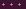= 0. I.e. [Q] ZP(α). Hence. we know that f([Q]) = 0. But by (2) again, this means that f(Q) = 0. CASE 1 DONE.
CASE 2: Q = 0:
One thing that was initially very confusing to me was why the deg f > 0 assumption is necessary. It's not in the original Nullstellensatz, so why is it included here? This case explains why. We can't convert Q to a projective point like in case 1 because Q = 0. BUT, since f is assumed to be homogenous with positive degree, f(Q) = 0 automatically. So there it is. CASE 2 DONE.

What have we shown? We've shown that for any Q ZA(α),f(Q) = 0. I.e. we've satsified the conditions of the regular Nullstellensatz. Hence, fq α for some q > 0. So we're done.